# VBA String Data Type (Dim Variable)

## String Variable Type

The String data type is one of the most common data types in VBA. It stores “strings” of text.

To declare an variable String variable, you use the Dim Statement (short for Dimension):

``Dim strName as String``

To assign a value to a variable, you use the equal sign:

``strName = "Fred Smith"``

Putting this in a procedure looks like this:

``````Sub strExample()
'declare the string
Dim strName as string
'populate the string
strName = "Fred Smith"
'show the message box
MsgBox strname
End Sub``````

If you run the code above, the following message box will be shown.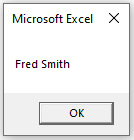### Fixed String Variable

There are actually 2 types of string variables – fixed and variable.

The “variable” string variable (shown in the previous example) allows your string to be any length. This is most common.

The “fixed” string variable defines the size of the string. A fixed string can hold up to 65,400 characters.

``Dim strName as string *20``

When you define a fixed variable, the number of characters in the variable is locked in place, even if you use fewer characters.

Notice the spaces in the graphic below – the variable has place holders for the rest of the characters in the string as ‘Fred Smith’ is less than 20 characters.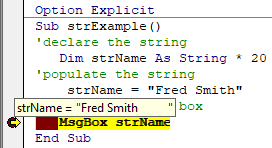However, if you have declared a string without specifying the length, then the string will only hold as many characters as are passed to it.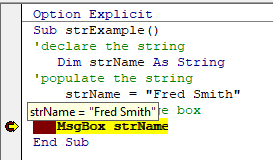## Declare String Variable at Module or Global Level

In the previous example, you declared the String variable within a procedure. Variables declared with a procedure can only be used within that procedure.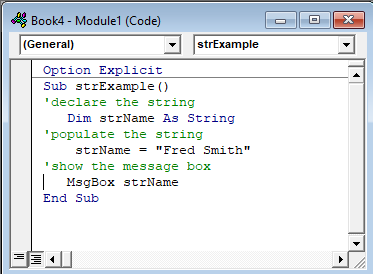Instead, you can declare String variables at the module or global level.

### Module Level

Module level variables are declared at the top of code modules with the Dim statement.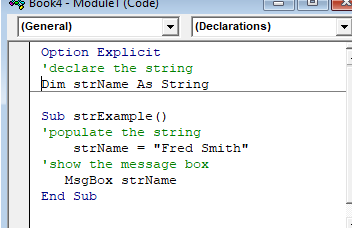These variables can be used with any procedure in that code module.

### Global Level

Global level variables are also declared at the top of code modules. However, instead of using the Dim statement, you use the Public statement to indicate that the string variable is available to be used throughout your VBA Project.

``Public strName as String``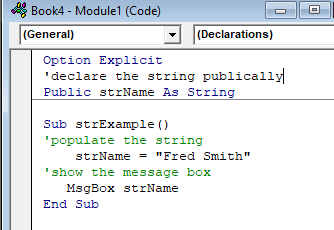If  you declared the string variable at a module level and used in a different module, an error would occur.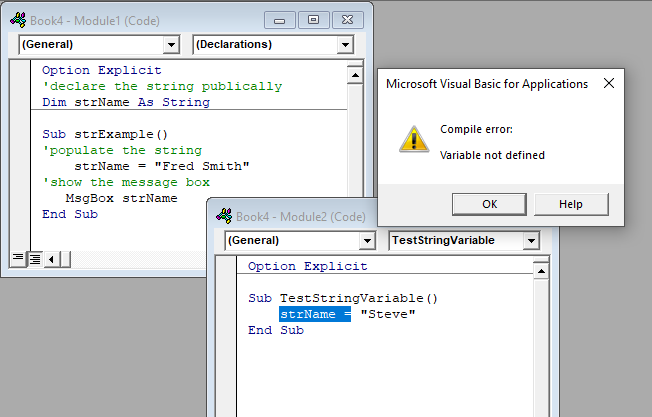However, if you use the Public keyword to declare the string variable, the error would not occur and the procedure would run perfectly.

## Convert Values stored as String

There may be a time when you have values in Excel that are stored as text – for example, you may have imported a CSV file which may have brought in text instead of numbers.

Note that the value in A1 is left-aligned, indicating a text value.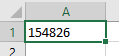You can use a VBA Function can be used to convert these numbers to text

``````Sub ConvertValue()
'populate the string
strQty = Range("A1")
'populate the double with the string
dblQty = strQty
'populate the range with the number
Range("A1") = dblQty
End Sub``````

Once you run the code, the number will move to the right-indicating that it is now stored as a number.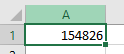This is particularly useful when you loop through a large range of cells.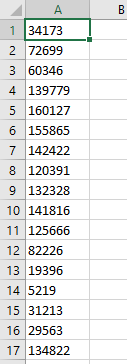``````Sub ConvertValue()
Dim strQty As String, dblQty As Double
Dim rw As Integer, i As Integer
'count the rows to convert
rw = Range("A1", Range("A1").End(xlDown)).Rows.Count
'loop through the cells and convert each one to a number
For i = 0 To rw – 1
'populate the string
strQty = Range("A1").Offset(i, 0)
'populate the double with the string
dblQty = strQty
'populate the range with the number
Range("A1").Offset(i, 0) = dblQty
Next i
End Sub``````

The result will be that all the cells are then converted to numbers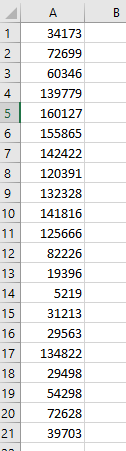Stop searching for VBA code online. Learn more about AutoMacro - A VBA Code Builder that allows beginners to code procedures from scratch with minimal coding knowledge and with many time-saving features for all users!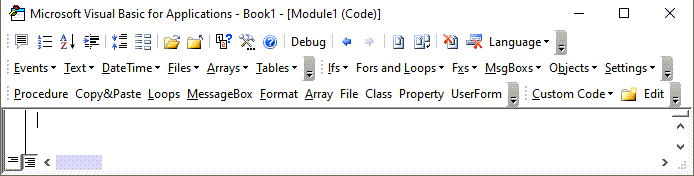## Convert String Stored as Values

Similarly, there might be values that you need to convert from a string to a value – for example, if you require a leading zero on a telephone number.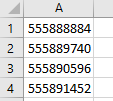``````Sub ConvertString()
Dim strPhone As String, dblPhone As Double
Dim rw As Integer, i As Integer
'count the rows to convert
rw = Range("A1", Range("A1").End(xlDown)).Rows.Count
'loop through the cells and convert each one to a number
For i = 0 To rw – 1
'populate the string
dblPhone = Range("A1").Offset(i, 0)
'populate the double with the string
strPhone = "'0" & dblPhone
'populate the range with the number
Range("A1").Offset(i, 0) = strphone
Next i
End Sub``````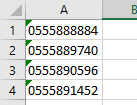Note that you need to  begin the text string with an apostrophe (‘), before the zero in order to tell Excel to enter the value as string.